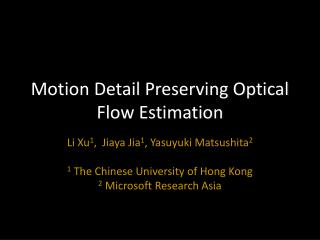DownloadDownload PresentationMotion Detail Preserving Optical Flow Estimation

# Motion Detail Preserving Optical Flow Estimation

Download Presentation## Motion Detail Preserving Optical Flow Estimation

- - - - - - - - - - - - - - - - - - - - - - - - - - - E N D - - - - - - - - - - - - - - - - - - - - - - - - - - -
##### Presentation Transcript

1. Motion Detail Preserving Optical Flow Estimation Li Xu1, Jiaya Jia1, Yasuyuki Matsushita2 1 The Chinese University of Hong Kong 2 Microsoft Research Asia

2. Conventional Optical Flow • Middlebury Benchmark [Baker et al. 07] • Dominant Scheme: Coarse-to-Fine Warping

3. Large Displacement Optical Flow • Region Matching [Broxet al. 09, 10] • Discrete Local Search [Steinbruckeret al. 09]

4. Both Large and Small Motion Exist • Capture large motion • Preserve sub-pixel accuracy

5. Our Work • Framework • Extended coarse-to-fine motion estimation for both large and small displacement optical flow • Model • A new data term to selectively combine constraints • Solver • Efficient numerical solver for discrete-continuous optimization

6. Outline • Framework • Extended coarse-to-fine motion estimation for both large and small displacement optical flow • Model • A new data term to selectively combine constraints • Solver • Efficient numerical solver for discrete-continuous optimization

7. The Multi-scale Problem

8. The Multi-scale Problem Ground truth Ground truth Ground truth

9. The Multi-scale Problem Ground truth Ground truth Ground truth

10. Ground truth Ground truth Estimate Estimate Estimate … Ground truth

11. The Multi-scale Problem • Large discrepancy between initial values and optimal motion vectors • Our solution • Improve flow initialization to reduce the reliance on the initialization from coarser levels

12. Extended Flow Initialization • Sparse feature matching for each level

13. Extended Flow Initialization • Identify missing motion vectors

14. Extended Flow Initialization • Identify missing motion vectors

15. Outline • Framework: extended initialization for coarse-to-fine motion estimation • Model: selective data term • Efficient numerical solver

16. Data Constraints • Average • Color constancy • Gradient constancy

17. Problems • Pixels moving out of shadow • Color constancy is violated : ground truth motion of p1 • Gradient constancy holds • Average:

18. Problems • Pixels undergoing rotational motion • Color constancy holds : ground truth motion of p2 • Gradient constancy is violated • Average:

19. Our Proposal • Selectively combine the constraints where

20. Comparisons

21. Outline • Framework: extended initialization for coarse to fine motion estimation • Model: selective data term • Efficient numerical solver

22. Energy Functions and Solver • Total energy • Probability of a particular state of the system

23. Mean Field Approximation • Partition function • Sum over all possible values of α . . . The effective potential Eeff (u)[Geiger & Girosi, 1989]

24. Optimal condition (Euler-Lagrange equations) • It decomposes to {

25. {

26. Algorithm Skeleton { • For each level • Extended Flow Initialization (QPBO) • Continuous Minimization (Iterative reweight) • Update • Compute flow field (Variable Splitting)

27. Results Difference Ours Averaging constraints

28. Middlebury Dataset EPE=0.74

29. Results from Different Steps Coarse-to-fine Extended coarse-to-fine

30. EPE=0.15 rank =1 EPE=0.24 rank =1

31. Large Displacement Overlaid Input

32. Large Displacement • Motion Estimates Coarse-to-fine Our Result Warping Result

33. Comparison • Motion Magnitude Maps LDOP [Brox et al. 09 ] [Steinbrucker et al. 09] Ours

34. More Results Overlaid Input

35. Our Result Conventional Coarse-to-fine

36. More Results Overlaid Input

37. Our Result Coarse-to-fine

38. Conclusion • Extended initialization (Framework) • Selective data term (Model) • Efficient numerical scheme (Solver) • Limitations • Featureless motion details • Large occlusions

39. Thank you!

40. More Results Overlaid Input

41. Our Results Coarse-to-fine